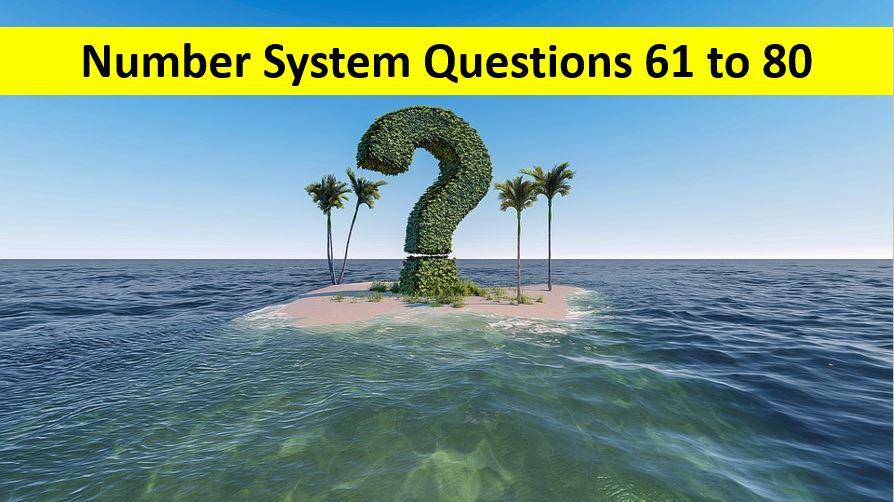Monday, June 1, 2020
Home > Latest Announcement > Number System Questions With Solutions Q No 61 To Q No 80

# Number System Questions With Solutions Q No 61 To Q No 80# Number System Questions With Solutions Q No 61 to Q No 80

In this post, you will get some important Number System Questions with Solutions Q No 61 To Q No 80. Questions will be solved soon.

If you can solve these Number Systems Question with Solutions Q No 61 To Q No 80. then please send us the solutions on my contact email: amansmathsblogs@gmail.com.

Ques No 61:

A two digit number N contains the smaller of the two digits in the unit place. The product of the digits is 40 and the difference between the digits is 3. The sum of the digits in N is

Options:

A. 11

B. 12

C. 13

D. 14

Solution:

Ques No 62:

The smallest prime number that divides the sum (711 + 1113) is

Options:

A. 2

B. 3

C. 5

D. 7

Solution:

Ques No 63:

How many 3-digit numbers do not have an even digit or a zero?

Options:

A. 60

B. 180

C. 125

D. 150

Solution:

Ques No 64:

The number 3072 is divisible by both 6 and 8. Which one of the following is the first integer larger than 3072 that is also divisible by both 6 and 8?

Options:

A. 3084

B. 3086

C. 3090

D. 3096

Solution:

Ques No 65:

In the 5-Digit number a6a41 each of the a’s represent the same digit. If the is divisible by 9, then the digit represented by ‘a’ is

Options:

A. 3

B. 7

C. 6

D. 8

Solution:

Ques No 66:

If n is a perfect square, then the next perfect square greater than n is

Options:

A. n2 + 1

B. n2 + n

C. n + 2+ 1

D. 2n + 1

Solution:

Ques No 67:

Which one of the following is an odd number?

Options:

A. 20012 + 3

B. 20023 + 10

C. 20032 + 7

D. 20043 + 1

Solution:

Ques No 68:

When the repeating decimal 0.45454545….. is written is simplest fractional from, the sum of the numerator and denominator is

Options:

A. 5

B. 11

C. 55

D. 16

Solution:

Ques No 69:

One hundred monkeys have 100 apples to divide. Each adult gets three apples while three children share one. Number of adult monkeys are:

Options:

A. 20

B. 25

C. 30

D. 33

Solution:

Ques No 70:

The remainder when 784 is divided by 342 is

Options:

A. 0

B. 1

C. 49

D. 214

Solution:

Ques No 71:

What is the remainder left after dividing 1!+2!+3!…..+100! by 7?

Options:

A. 0

B. 5

C. 2

D. 4

Solution:

Ques No 72:

The highest power of 9 dividing 99! completely is

Options:

A. 12

B. 14

C. 22

D. 24

Solution:

Ques No 73:

The number 3 divides ‘a’ with a result of ‘b’ and a remainder of 2. The number 3 divides ‘b’ with a result of 2 and a remainder of 1. What is the value of a?

Options:

A. 13

B. 17

C. 21

D. 23

Solution:

Ques No 74:

Number of zeros in the product of 5 x 10 x 25 x 40 x 50 x 55 x 65 x 125 x 80, is

Options:

A. 8

B. 9

C. 12

D. 13

Solution:

Ques No 75:

A farmer divides his herd of x cows among his 4 son’s such that first son gets one-half of the herd, the second son gets one fourth, the third son gets one-fifth and the fourth son gets 7 cows, then the value of x is:

Options:

A. 100

B. 140

C. 160

D. 180

Solution:

Ques No 76:

When a particular positive number is divided by 5, the remainder is 2. If the same number is divided by 6, the remainder is 1. If the difference between the quotients of division is 3, then find the number

Options:

A. 37

B. 97

C. 67

D. 87

Solution:

Ques No 77:

It is given that 232+1 is exactly divisible by a certain number. Which one of the following is also divisible by the same number?

Options:

A. 296+1

B. 216+1

C. 28+1

D. 264+1

Solution:

Ques No 78:

What is the last digit of the number 3^5^7^9+1?

Options:

A. 1

B. 3

C. 4

D. 7

Solution:

Ques No 79:

If x and y are any natural numbers, then which of the following is an odd number?

Options:

A. xy + yx + (x – y)(xy + x)

B. xy(x + y)(xy + x)

C. yx + (xy – y)(xy – x)

D. None of these

Solution:

Ques No 80:

A and B are playing mathematical puzzles. A asks B “which whole numbers, greater than one, can divide all the nine three digit numbers 111, 222, 333, 444, 555, 666, 777, 888 and 999?” B immediately gave the desired answer. It was

Options:

A. 3, 37, 119

B. 3, 37, 111

C. 9, 37, 111

D. 3, 9, 37

Solution:

error: Content is protected !!